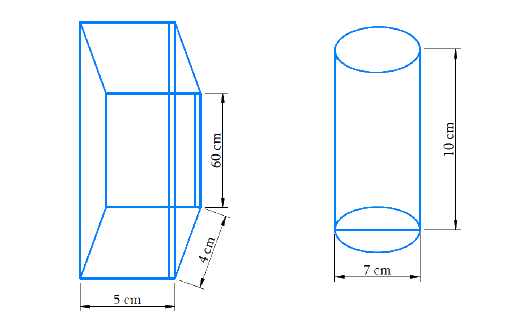# Ex.13.6 Q3 Surface Areas and Volumes Solution - NCERT Maths Class 9

## Question

A soft drink is available in two packs – (i) a tin can with a rectangular base of length $$5 \;cm$$ and width $$4\; cm$$, having a height of $$15\ cm$$ and (ii) a plastic cylinder with circular base of diameter $$7\ cm$$ and height $$10\ cm$$. Which container has greater capacity and by how much?

Video Solution
Surface-Areas-And-Volumes
Ex exercise-13-6 | Question 3

## Text Solution

Reasoning:

Volume of the cuboid is $$lbh$$ and volume of cylinder in\begin{align}\,\pi {r^2}h \end{align}

What is known?

Measurements of cuboid can. Measurements of cylindrical can.

What is unknown?

Which can is big and how much ?

Steps:

DiagramTin Can:

$$\text{Length} \; (l) = 5\ cm$$

$$\text{Breadth}\; (b) = 4\ cm$$

$$\text{Height}\; (h) = 15\ cm$$

$$\text{Capacity = Volume} = l b h$$.

\begin{align}&= 5 \times 4 \times 15 \\&= 300\,\,c{m^3} \\ \end{align}

Plastic Cylinder:

$$\text{Diameter} (2r) =7\; cm$$

Radius\begin{align} \,(r) = \frac{7}{2} \end{align}cm

$$\text{Height}\; (h) =10\; cm$$

\begin{align}\text{Capacity} &= \rm{Volume} = \pi {r^2}h\ \\ &= \frac{{22}}{7} \times \frac{7}{2} \times \frac{7}{2} \times 10\\ &= 385\,\,\,c{m^3} \end{align}

Clearly, the plastic cylinder has greater capacity than the tin container.

Difference\begin{align} = \,385 - 300 = 85\,c{m^3} \end{align}

Answer:

The plastic cylindrical can have more capacity than the Tin Can by\begin{align}\, = 85\,\,c{m^3} \end{align}.

Learn from the best math teachers and top your exams

• Live one on one classroom and doubt clearing
• Practice worksheets in and after class for conceptual clarity
• Personalized curriculum to keep up with school